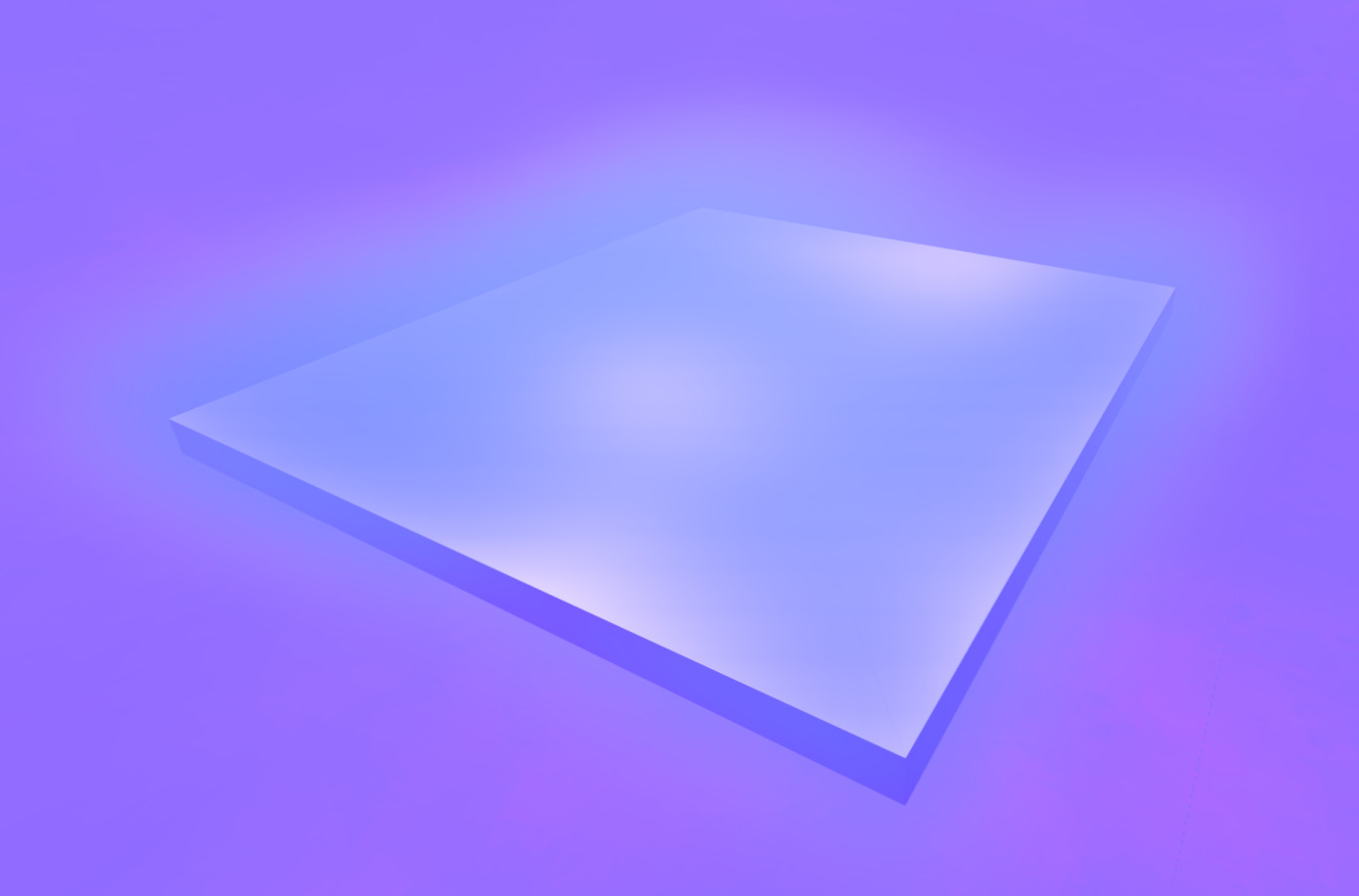# Moving gradient noise fog/ mist for Godot 4

Fog shader that calculates gradient noise in 3D in three octaves that are moving in different directions and speeds. Makes for a nice atmospheric mist or ground fog. Implemented based on clayjohn’s issue comments on the fog shaders and Inigo Quilez’s 3D gradient noise from ShaderToy (under MIT license).

Just attach this to a FogVolume of the desired size and adjust the `fade_out_height` to the global coordinates of your volume (in case you don’t need this you can comment out line 25).

Tested in Godot 4.0 stable.

``````shader_type fog;

uniform float base_density: hint_range(0, 1) = 0.8;
uniform vec3 base_color: source_color;

uniform vec3 noise_amounts = vec3(0.4, 0.35, 0.25); // should add up to 1
uniform vec3 noise_scales = vec3(1.0, 2.0, 4.0);
uniform vec3 noise_velocity1 = vec3(0.5, 0.0, 0.0);
uniform vec3 noise_velocity2 = vec3(1, 0.0, -1);
uniform vec3 noise_velocity3 = vec3(0.0, 0.6, 0.3);

void fog() {
vec3 base_pos = WORLD_POSITION * vec3(0.1, 0.5, 0.1);
DENSITY = base_density;
float noise1 = noise(base_pos * noise_scales.x + TIME * noise_velocity1) * noise_amounts.x;
float noise2 = noise(base_pos * noise_scales.y + TIME * noise_velocity2) * noise_amounts.y;
float noise3 = noise(base_pos * noise_scales.z + TIME * noise_velocity3) * noise_amounts.z;
DENSITY *= smoothstep(-0.1, 0.0, noise1 + noise2 + noise3);
ALBEDO = base_color;
}

// Permission is hereby granted, free of charge, to any person obtaining a copy of this software and associated documentation files (the "Software"), to deal in the Software without restriction, including without limitation the rights to use, copy, modify, merge, publish, distribute, sublicense, and/or sell copies of the Software, and to permit persons to whom the Software is furnished to do so, subject to the following conditions: The above copyright notice and this permission notice shall be included in all copies or substantial portions of the Software. THE SOFTWARE IS PROVIDED "AS IS", WITHOUT WARRANTY OF ANY KIND, EXPRESS OR IMPLIED, INCLUDING BUT NOT LIMITED TO THE WARRANTIES OF MERCHANTABILITY, FITNESS FOR A PARTICULAR PURPOSE AND NONINFRINGEMENT. IN NO EVENT SHALL THE AUTHORS OR COPYRIGHT HOLDERS BE LIABLE FOR ANY CLAIM, DAMAGES OR OTHER LIABILITY, WHETHER IN AN ACTION OF CONTRACT, TORT OR OTHERWISE, ARISING FROM, OUT OF OR IN CONNECTION WITH THE SOFTWARE OR THE USE OR OTHER DEALINGS IN THE SOFTWARE.

// All noise functions here:
//

vec3 hash(vec3 p) {
p = vec3(dot(p,vec3(127.1,311.7, 74.7)),
dot(p,vec3(269.5,183.3,246.1)),
dot(p,vec3(113.5,271.9,124.6)));

return -1.0 + 2.0 * fract(sin(p) * 43758.5453123);
}

float noise(in vec3 p) {
vec3 i = floor(p);
vec3 f = fract(p);

vec3 u = f*f*(3.0-2.0*f);

return mix( mix( mix( dot( hash( i + vec3(0.0,0.0,0.0) ), f - vec3(0.0,0.0,0.0) ),
dot( hash( i + vec3(1.0,0.0,0.0) ), f - vec3(1.0,0.0,0.0) ), u.x),
mix( dot( hash( i + vec3(0.0,1.0,0.0) ), f - vec3(0.0,1.0,0.0) ),
dot( hash( i + vec3(1.0,1.0,0.0) ), f - vec3(1.0,1.0,0.0) ), u.x), u.y),
mix( mix( dot( hash( i + vec3(0.0,0.0,1.0) ), f - vec3(0.0,0.0,1.0) ),
dot( hash( i + vec3(1.0,0.0,1.0) ), f - vec3(1.0,0.0,1.0) ), u.x),
mix( dot( hash( i + vec3(0.0,1.0,1.0) ), f - vec3(0.0,1.0,1.0) ),
dot( hash( i + vec3(1.0,1.0,1.0) ), f - vec3(1.0,1.0,1.0) ), u.x), u.y), u.z );
}``````
###### Tags
animated, atmospheric, Fog, mistThe shader code and all code snippets in this post are under MIT license and can be used freely. Images and videos, and assets depicted in those, do not fall under this license. For more info, see our License terms.

### 2D Fog Overlay

Subscribe
Notify ofInline FeedbacksIliya
6 months ago

Too many arguments for “noise()” call. Expected at most 0 but received 1. Godot 4 stable.Nevermore
6 months ago